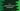# C++ getchar() function explanation with example## C++ getchar() function :

C++ provides one function called getchar() to read user inputs.This function reads the next character from stdin. In this post, we will learn how this function works with an example.

### getchar() syntax :

The syntax of the getchar() function is as below :

``````int getchar ( void );
``````

### Parameters and return types :

This function doesn’t take any parameter.

On success, it returns one integer value.

On failure, it returns EOF. Note that if it reads end-of-file, EOF is returned and sets the stdin eof indicator. For error or failure, EOF is returned and sets the stdin error indicator. EOF is returned in both cases.

This is actually a C library used for input/output operations. It is defined in the _cstdio _(C standard input-output library) header of C++.

### Example of getchar() :

Let me show you one simple example of how getchar works :

``````#include <iostream>
#include <cstdio>
using namespace std;

int main()
{
int character;

cout << "Enter a string :" << endl;

do
{
character = getchar();
cout << "Reading .." << character << " or " << char(character) << endl;
} while (character != '\n');
return 0;
}
``````

### Explanation :

In this example,

• character is an integer variable.

• At first, we are asking the user to enter a string.

• Next, we are reading the characters one by one using a do while loop and getchar() function.

• The print statement inside the do while loop prints the integer character value returned by the getchar function and also its character representation char(character).

### Sample Example :

``````Enter a string :
hello world